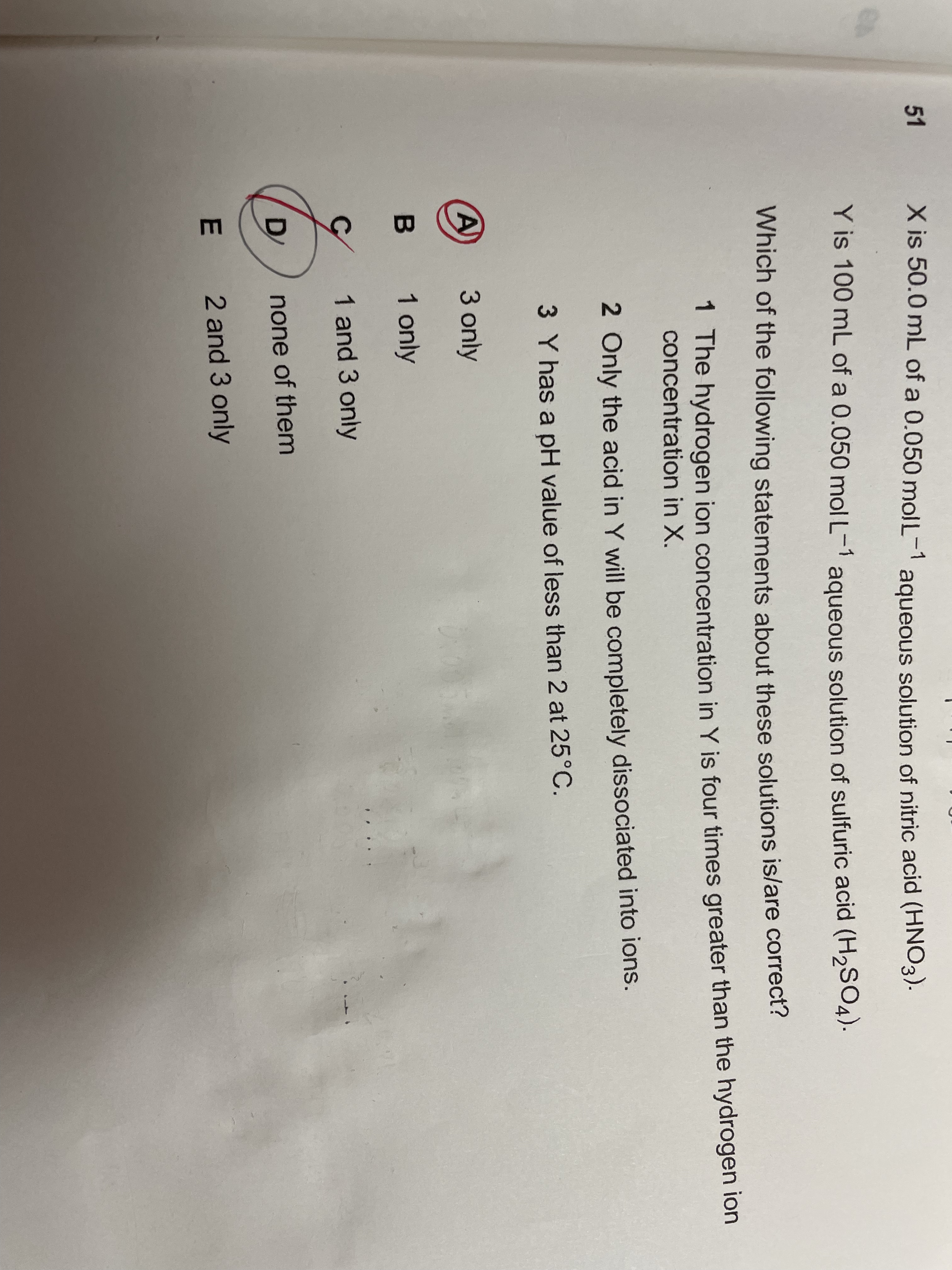# How can I solve this question? ; Chemistry Q from IMAT 2021.

I’ve tried so many times for figuring out this question but still couldn’t.
Is there anyone who is willing to explain to me the method of solving this question since I couldn’t find any materials that explain it?
And why would option 1 be incorrect?1 Like

Hi!
In each mol of HNO3 there is 2 moles of H atom. Since there is 5×10^-2 mol/lit and 50ml of HNO3 solution, we can find out that there is 5×0.01×0.05=0.0025 mol of HNO3, so the concentration of H atom will be 0.0025÷0.05=0.05
In each mole of H2SO4 there is 2 moles of H atom, H2SO4 is 0.05mol/lit and 100ml.
So the mole of H2SO4 is 0.005, and the mole of H atom is 0.005×2=0.01, and the concentration of H atom is 0.01÷0.1=0.1
Concentration of H in H2So4/ concentration of H in HNO3 = 0.1÷0.05=2 . So option 1 is wrong , HNO3 is a strong acid to! So it can be completely dissociated into ions, option 2 is wrong.
The PH of H2SO4 is -log0.05=-(-2+0.7)=1.3
So the PH is less than 2.

2 Likes

I’ve got 2 more questions while solving it by following your solution which are:
1)Why do you have to multiply 0.0025mol by 0.05L further more to get the [H+]? (in the example of X)
I know that’s how we can calculate the concentration but from my textbook so my understanding, the formula of [H+] is:
[H+]= Concentration × Valency × Degree of ionization.
If I solve it based on this formula, It’ll be 0.0025×1 ×1= 0.0025 and is the same case for Y solution.
So this is a confusing point to me….

1. For option 3, why do you insert 0.05 instead of 0.005 or 0.1 which we’ve calculated in the option 1 since the PH formula demonstrates “pH=-log[H+].
So I thought I had to insert either 0.005 or 0.01 not 0.05.

It’s a long sentence but it would be very very thankful if you can explain these 2 questions:)

1 Like

Hey!
Anytime!

1. I didn’t multiply it, I divided it: 0.0025÷0.05=0.05 cause 0.0025 was the mole and 0.05 was the volume of the solution; I did it so as to find the concentration of the H atom.
You didn’t pay attention to the fact that 0.0025 is the mole, not the concentration!

2)0.005 is the mole of H2SO4, not the concentration. We need to divide it by 0.1 lit to find the concentration of the solution.
0.005÷0.1=0.05

This picture may help!

2 Likes